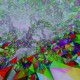# Gravity matters

John Barrett's research website

with one comment

Spin foam models of quantum gravity rely heavily on the use of spin networks, so it is a good idea to understand spin networks before trying to read about spin foam. There isn’t a comprehensive book on either spin foam models or on spin networks. For spin foams, that’s maybe because the subject is still actively evolving. But for spin networks, there could be but no-one has written it yet.

A good introduction to spin networks is Seth Major’s A Spin Network Primer, in which diagrams stand for tensor calculations. Another key reference is the 1983 thesis of John Moussouris, Quantum Models of Space-Time Based on Recoupling Theory. See also my spin foam lectures.

A more abstract approach is the skein theory approach which does the whole theory using the relations between the diagrams instead of tensor calculations. This is the original approach of Penrose in Angular momentum: an approach to combinatorial space-time, and other papers, available here. The theory was made more systematic when quantum groups were invented, and is neatly summarised in chapters 1-9 of the book of Kauffman and Lins, Temperley-Lieb Recoupling Theory and Invariants of 3-Manifolds. It is a generalisation of the original spin networks due to the introduction of a parameter A. The connection with tensor calculations is described in Kauffman’s Spin Networks And The Bracket Polynomial. To recover the Penrose/Seth Major approach, one should put A=-1 everywhere.

Spin networks lead naturally to the gold standard model of quantum gravity, the Ponzano-Regge model.  This is a model for  three-dimensional space-time. It is covered in my paper with Ileana Naish-Guzman called The Ponzano-Regge model, and also in John Moussouris’ thesis. It is worth getting hold of the original paper, Semiclassical limit of Racah coefficients by Ponzano and Regge as it has a wealth of ideas and calculations, as well as being a useful reference for the precise definition of a 6j-symbol. The Ponzano-Regge paper does not quite give a general definition of the Ponzano-Regge model, because the authors were not clear about the correct sign factors. This problem has frequently been overlooked in the intervening years, but is sorted out in my paper with Ileana.

The quantum group cousin of Ponzano-Regge is the Turaev-Viro model, in which the additional parameter A can have a range of values and determines the value of the cosmological constant. This model is fully defined in a mathematical sense (unlike the Ponzano-Regge model which sometimes has infinities). It is therefore a better starting point for more mathematical investigations of 3d gravity. It is introduced in my spin foam lectures. The starting point for the literature on observables and particle couplings is my paper Geometrical measurements in three-dimensional quantum gravity.

Another generalisation of spin networks is to use the diagrams in a space-time as observables in a gauge theory. The edges of the diagram are parallel transport operators. This is much used in loop quantum gravity, where the idea is to rewrite general relativity as a gauge theory. The approach is explained in detail in Carlo Rovelli’s book Quantum Gravity.

I should warn that the original idea of loop quantum gravity, as a quantization of this gauge theory, faces obstacles and is not currently a promising research direction. However the basic ideas are often used to motivate the closely-related spin foam models, as explained both by Rovelli’s Living Review and by Etera Livine. Some further reading material for spin foam models is found on Dan Christensen’s page.

Written by johnwbarrett

15 December 2011 at 17:10

### One Response